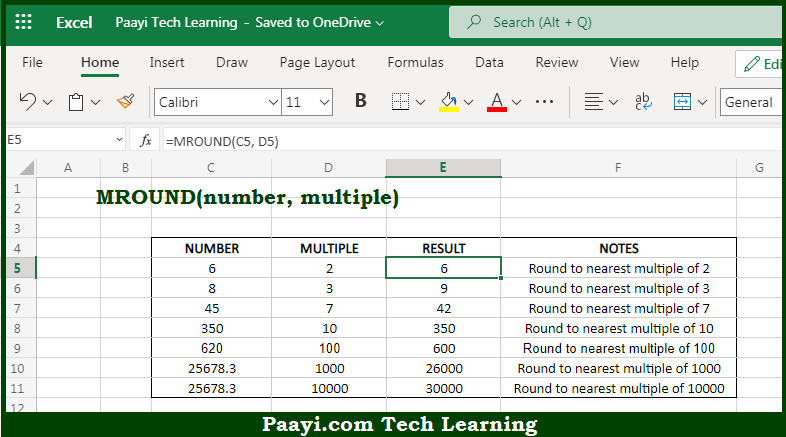# Learn How to Use Microsoft Excel MROUND Function

Written by | 0 Comments | 490 Views

In this article, you will learn how to use the Microsoft Excel MROUND function and its prime function in Microsoft Excel. You will also get to know the Microsoft Excel MROUND function return value and syntax with the help of some examples.

Microsoft Excel MROUND Function

The main function of the Microsoft Excel MROUND function is to round a number to the nearest specified multiple. That implies, with the help of MROUND function you can able to return the number rounded to the nearest multiple. It should be noted that MROUND will round the number up or down, that depends upon the nearest multiple. So, with the help of MROUND function, you can able to get the number rounded to the nearest multiple.

Return Value of MROUND Function

The return value will be the rounded number.

Syntax of MROUND Function

=MROUND(number, divisor)

Where the arguments:

• number: This is the number to be divided with.
• divisor: This is the number to divide with.

## How to Use Microsoft Excel MROUND Function?So we know that Microsoft Excel MROUND function you can able to round a number to the nearest specified multiple. That implies, with the help of MROUND function you can able to return the number rounded to the nearest multiple. It should be noted that MROUND will round the number up or down, that depends upon the nearest multiple. So, with the help of MROUND function, you can able to get the number rounded to the nearest multiple.